AVL树的插入与删除（详解）

# 一、AVL树的插入

public class BSTNode implements BalanceFactor {
private int data;
private int bf;
private BSTNode lchild, rchild, parent;

public BSTNode(int data) {// 构造一个结点
this.data = data;
this.bf = EH;//插入的结点平衡因子为0（EH）
this.lchild = null;
this.rchild = null;
this.parent = null;
}

public int getData() {
return data;
}

public void setData(int data) {
this.data = data;
}

public int getBf() {
return bf;
}

public void setBf(int bf) {
this.bf = bf;
}

public BSTNode getLchild() {
return lchild;
}

public void setLchild(BSTNode lchild) {
this.lchild = lchild;
}

public BSTNode getRchild() {
return rchild;
}

public void setRchild(BSTNode rchild) {
this.rchild = rchild;
}

public BSTNode getParent() {
return parent;
}

public void setParent(BSTNode parent) {
this.parent = parent;
}

}


public interface BalanceFactor {
public static final int LH = 1;// 左高
public static final int EH = 0;// 等高
public static final int RH = -1;// 右高
}


## 1.单右旋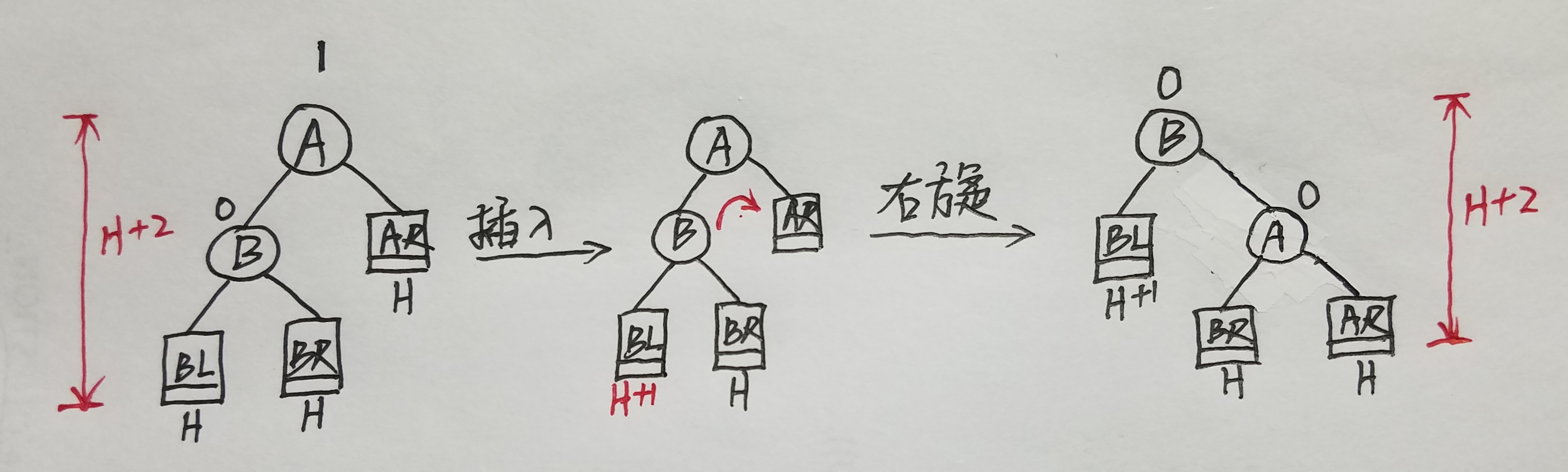单右旋的代码实现也很简单，单右旋的时候若H=0，需要注意，此时B.getRchild().setParent(A)会出错，因为得到的B点的右孩子为null。还有就是若A是根节点，A.getParent().setRchild()时也会报错。反正就是需要注意一下边界的情况。

	public void R_Rotate(BSTNode p) {// 以结点p右旋处理
BSTNode lc = p.getLchild();
lc.setParent(p.getParent());
if (p.getParent() != null) {
if (p.getParent().getLchild() == p) {// p是父结点的左孩子
p.getParent().setLchild(lc);
} else {// p是父结点的右孩子
p.getParent().setRchild(lc);
}
}
p.setLchild(lc.getRchild());
if (lc.getRchild() != null) {
lc.getRchild().setParent(p);
}
lc.setRchild(p);
p.setParent(lc);
if (lc.getParent() == null) {
root = lc;
}
}


## 2.先左后右旋转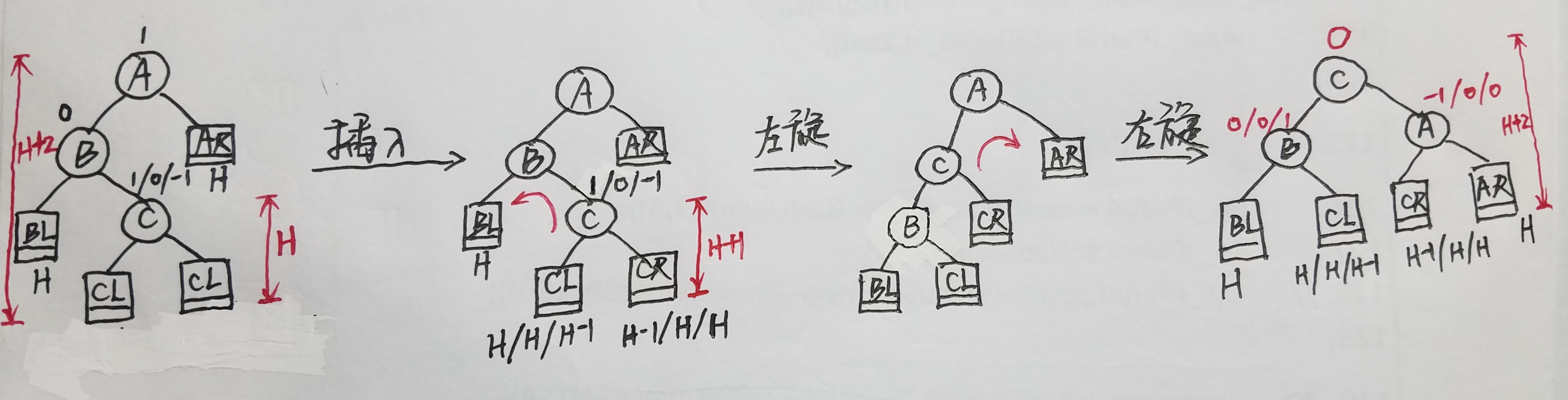无论先左后右还是先右后左旋转，都是由两个单旋转合成的，就是先对B左单旋，再对A右单旋就可以了，很简单的。

## 3.单左旋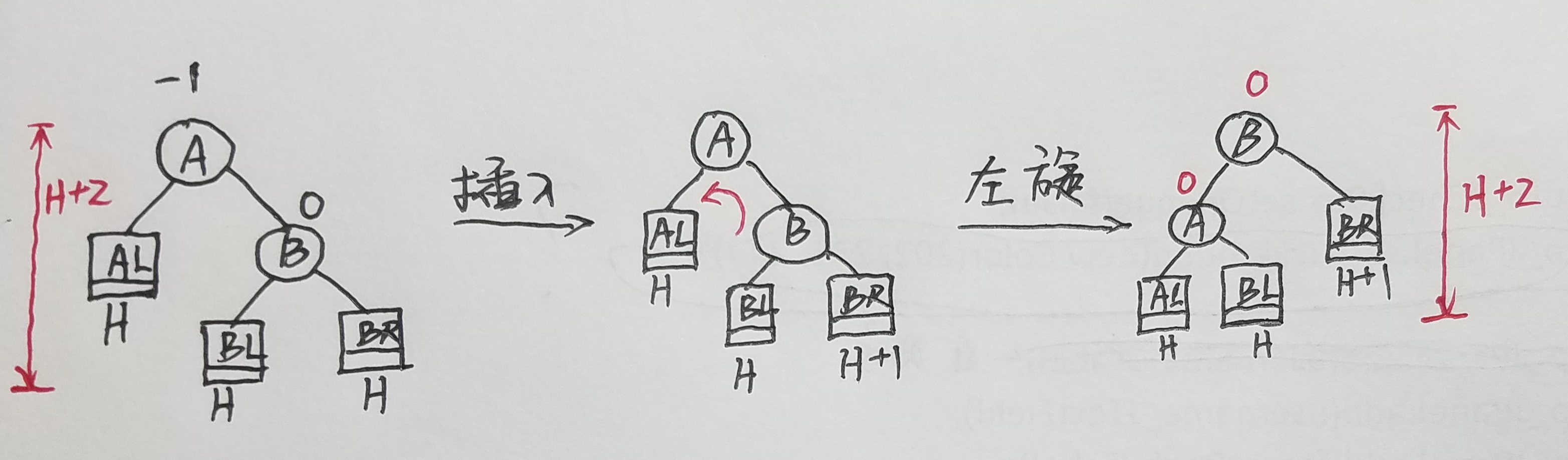public void L_Rotate(BSTNode p) {// 以结点p左旋处理
BSTNode lc = p.getRchild();
lc.setParent(p.getParent());
if (p.getParent() != null) {
if (p.getParent().getLchild() == p) {
p.getParent().setLchild(lc);
} else {
p.getParent().setRchild(lc);
}
}
p.setRchild(lc.getLchild());
if (lc.getLchild() != null) {
lc.getLchild().setParent(p);
}
lc.setLchild(p);
p.setParent(lc);
if (lc.getParent() == null) {// 如果旋转之后lc是根节点
root = lc;
}
}


## 4.先右后左双旋转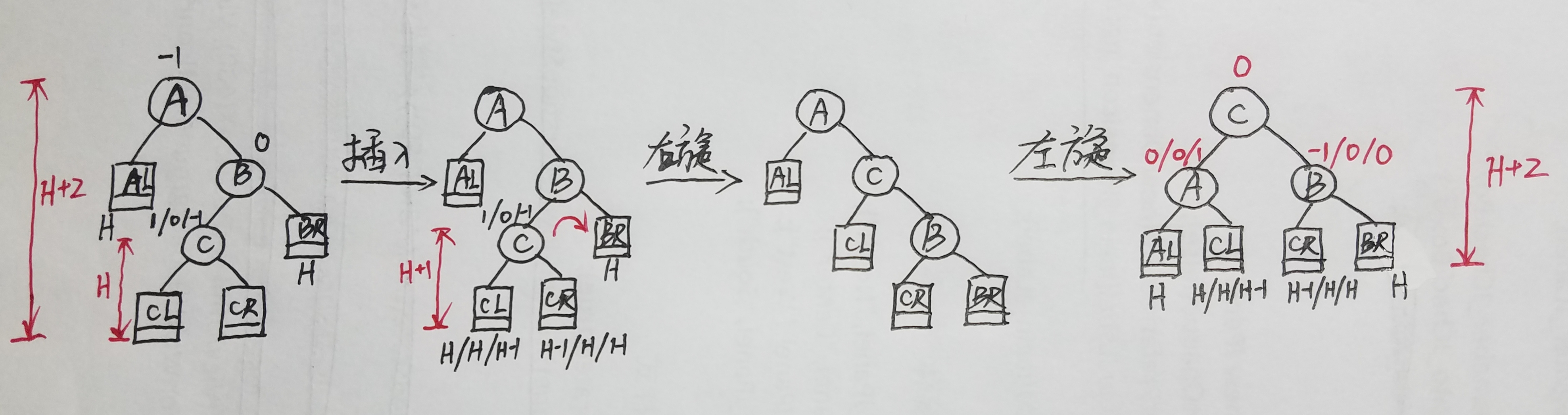插入的话需要旋转的就这四种情况。可能会有疑惑，为什么A平衡因子为0，B的平衡因子为±1的情况没有列出来?可以尝试自己画一下，这种情况的话是不需要旋转的，只是当前的树可能会变高。若这颗树变高了（假设当前变高的子树根节点为A），那么相对于这棵树的父节点来说，又会是属于上面的四种情况之一。

## 5.左右平衡处理

public void InLeftBalance(BSTNode T) {// 对以T为根节点的二叉树做左平衡处理(先改变结点的bf,再旋转)
BSTNode lc = T.getLchild();
switch (lc.getBf()) {
case LH:// 单右旋的
T.setBf(EH);
lc.setBf(EH);
R_Rotate(T);
break;
case RH:// 先左旋，再右旋的
BSTNode rd = lc.getRchild();
switch (rd.getBf()) {
case LH:
T.setBf(RH);
lc.setBf(EH);
break;
case EH:
T.setBf(EH);
lc.setBf(EH);
break;
case RH:
T.setBf(EH);
lc.setBf(LH);
break;
}
rd.setBf(EH);
L_Rotate(lc);//双旋转
R_Rotate(T);
}
}


public void InRightBalance(BSTNode T) {
BSTNode lc = T.getRchild();
switch (lc.getBf()) {
case RH:// 单左旋的
T.setBf(EH);
lc.setBf(EH);
L_Rotate(T);
break;
case LH:// 先右旋，再左旋的
BSTNode rd = lc.getLchild();
switch (rd.getBf()) {
case LH:
T.setBf(EH);
lc.setBf(RH);
break;
case EH:
T.setBf(EH);
lc.setBf(EH);
break;
case RH:
T.setBf(LH);
lc.setBf(EH);
break;
}
rd.setBf(EH);
R_Rotate(lc);
L_Rotate(T);
}
}


## 6.结点插入的实现

private static boolean taller;

	public boolean insertAVL(BSTNode T, int x) {// 以T为根节点的平衡二叉树中插入元素x，成功返回true,失败返回false,taller反应树是否长高
if (T == null) {// 只有刚开始插入树为空才会执行这一步
root = new BSTNode(x);
return true;
}
if (x == T.getData()) {// ①
taller = false;
return false;
} else if (x < T.getData()) {// ②
if (T.getLchild() == null) {// 检查左子树
BSTNode q = new BSTNode(x);
T.setLchild(q);// 直接插入
q.setParent(T);
switch (T.getBf()) {
case EH:
T.setBf(LH);
taller = true;
break;
case RH:
T.setBf(EH);
taller = false;
break;
}
return true;
}
if (!insertAVL(T.getLchild(), x)) {
return false;
}
if (taller) {
switch (T.getBf()) {// 检查结点的平衡因子
case LH:// 原本结点的左子树比右子树高，且在左子树中插入了，需要做左平衡处理,处理之后树的高度不变
InLeftBalance(T);
taller = false;
break;
case EH:// 左右子树同样高，在左子树中插入，只是树变高了、平衡因子变为1，但当前不用做平衡处理
T.setBf(LH);
taller = true;
break;
case RH:// 右子树比左子树高，在左子树中插入，树的高度不变，当前结点的平衡因子变为0
T.setBf(EH);
taller = false;
break;
}
}
} else {// ③
if (T.getRchild() == null) {// 检查右子树
BSTNode q = new BSTNode(x);
T.setRchild(q);
q.setParent(T);
switch (T.getBf()) {
case EH:
T.setBf(RH);
taller = true;
break;
case LH:
T.setBf(EH);
taller = false;
break;
}
return true;
}
if (!insertAVL(T.getRchild(), x)) {
return false;
}
if (taller) {
switch (T.getBf()) {
case LH:
T.setBf(EH);
taller = false;
break;
case EH:
T.setBf(RH);
taller = true;
break;
case RH:
InRightBalance(T);
taller = false;
break;
}
}
}
return true;
}


# 二、AVL树的删除

## 1.删除节点的分类

1.所删除的结点为叶子结点，可以将其直接删除，然后再依次向上调整使整棵树平衡。
2.删除的结点只有左子树且只有左子树一个节点（左右子树的高度差不超过1）。将待删除的结点与它的左子树结点值交换，删除叶子结点。这就转换成了第一种情况。

deleteAVL(T,x){
******
int value=T.getLchild().getDate();
deleteAVL(T,value);
T.setData(value);
******
}


3.删除的结点只有右子树且只有右子树一个节点，这样的情况以及转化方法和情况2几 乎是一样的，同理可以转化成情况1。
4.删除的节点左右子树都不空，这种情况和2.3的思想也是一样的。我们可以找到删除结点的前驱或者后继（中序遍历），前驱的位置是从该结点左转然后一直向右走到尽头（后继是从该节点右转然后向左走到尽头）。通过和2中类似的方法交换，将删除的结点与前驱结点元素值交换，再删除前驱结点。这里要删除的前驱位置结点可能会有左子树结点，那就再交换一次。情况四最多只需要两次交换。然后都能转化成情况1。这里可能看的有点迷糊，不过很正常，到时候看一下代码跟着代码走一次就全都明白了。

## 2.平衡旋转

###### （3）

1. B.getBf()=1，删除后经过旋转树的高度会减小。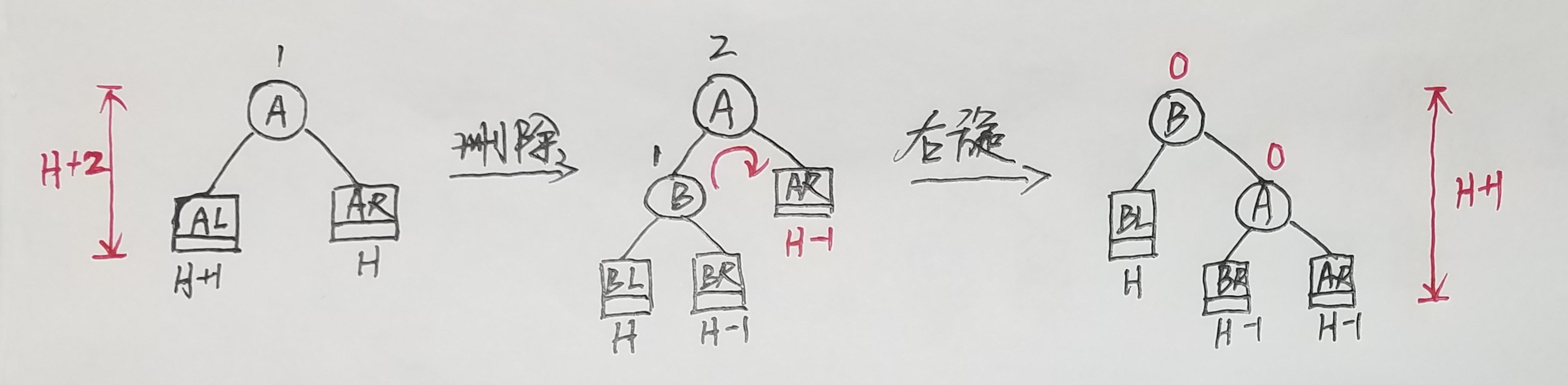2. B.getBf()=0，删除后经过旋转树的高度恢复为原来的高度。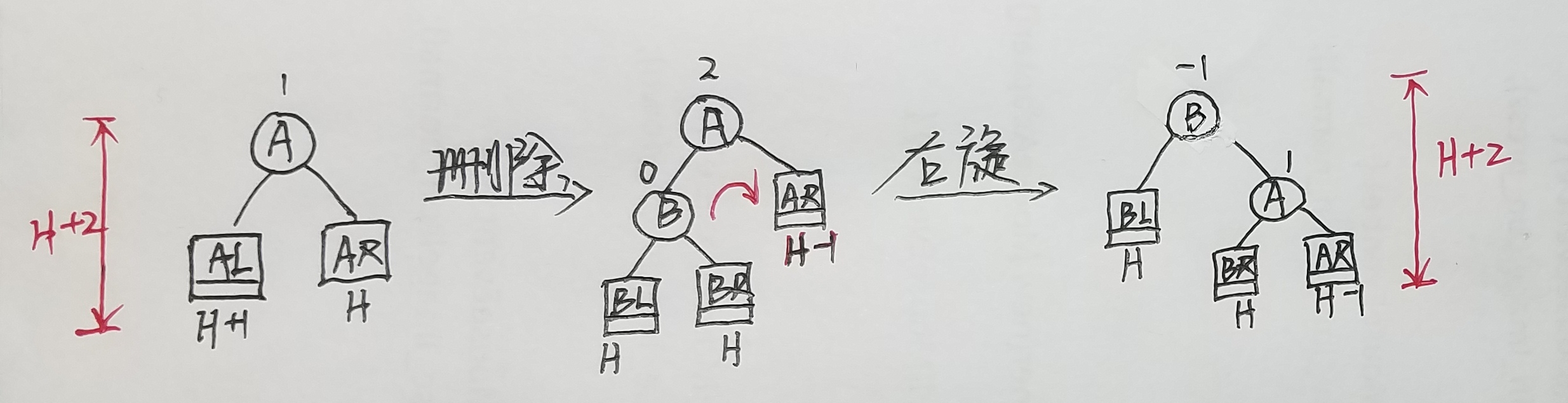3. B.getBf()=-1，删除后经过旋转树的高度会减小。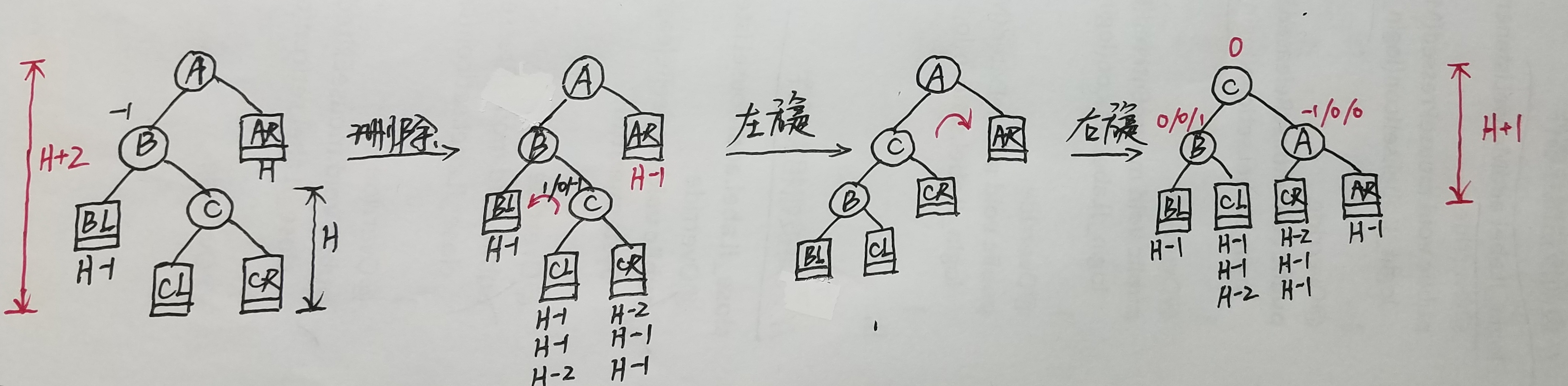###### （4）

1. B.getBf()=1，删除后经过旋转树的高度会减小。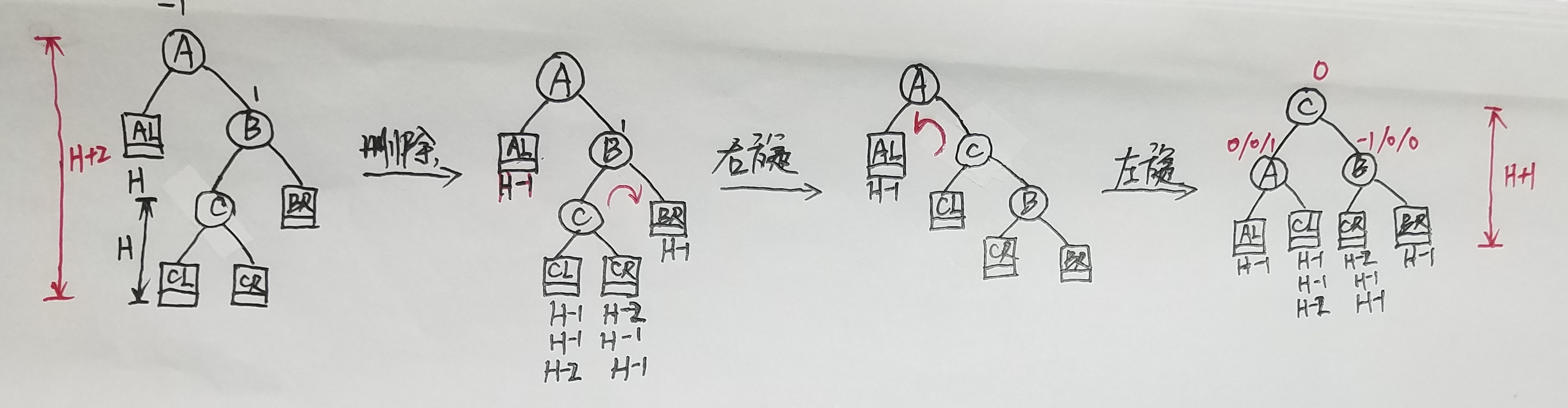2. B.getBf()=0，删除后经过旋转树的高度恢复为原来的。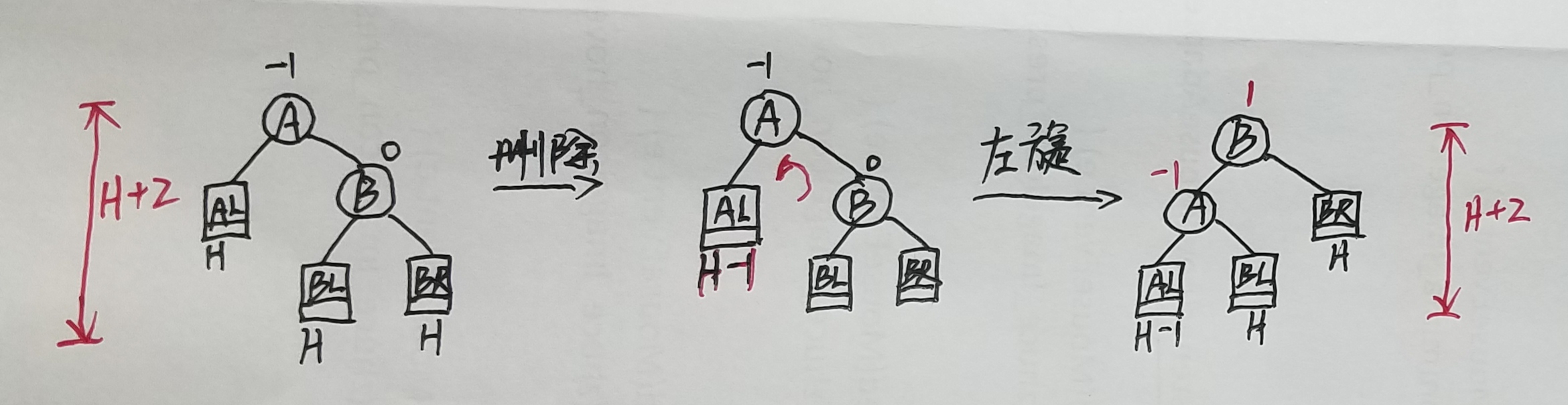3. B.getBf()=-1，删除后经过旋转树的高度会减小。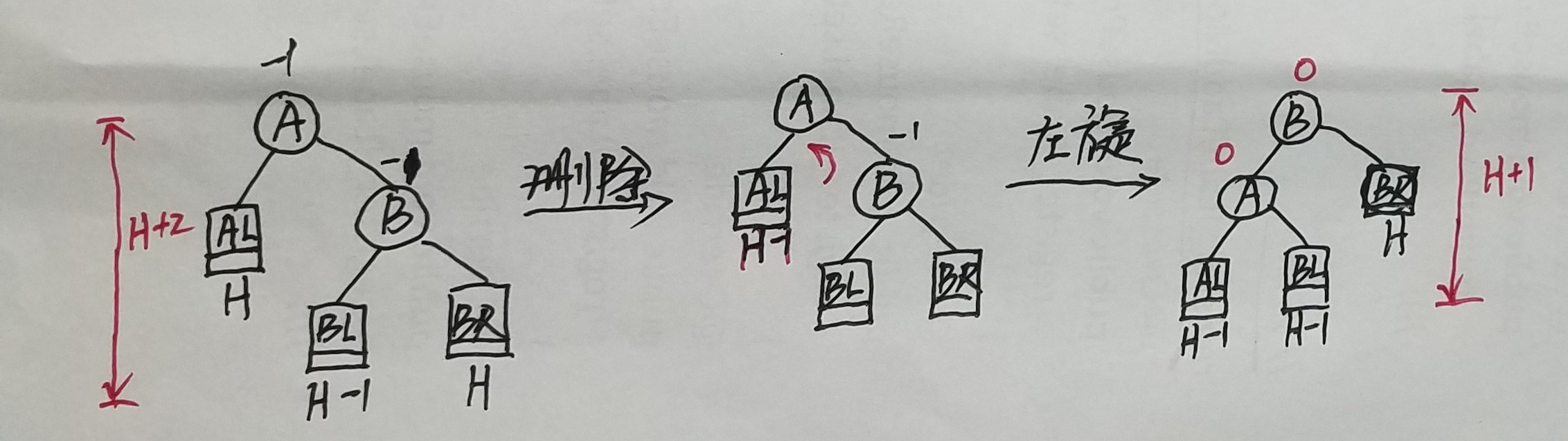## 3.左右平衡处理

public static boolean shorter;// 记录树是否变矮的标志，若shorter=true要进行相应的调整

public void DeLeftBalance(BSTNode T) {
// 删除结点导致以T的左孩子为根节点的树高度改变，T结点失衡，做左平衡处理
BSTNode p = T.getRchild();
switch (p.getBf()) {
case LH:
// 先改结点的BF值
BSTNode q = p.getLchild();
switch (q.getBf()) {
case LH:
T.setBf(EH);
p.setBf(RH);
break;
case EH:
T.setBf(EH);
p.setBf(EH);
break;
case RH:
T.setBf(LH);
p.setBf(EH);
break;
}
q.setBf(EH);
// 再先右-左旋
R_Rotate(p);
L_Rotate(T);
shorter = true;
break;
case EH:// 左旋
p.setBf(LH);
T.setBf(RH);
L_Rotate(T);
shorter = false;
break;
case RH:// 左旋
p.setBf(EH);
T.setBf(EH);
L_Rotate(T);
shorter = true;
break;
}
}

public void DeRightBalance(BSTNode T) {
// 删除结点导致以T的右侧孩子为根节点的树高度改变，T结点失衡，做右平衡处理
BSTNode p = T.getLchild();
switch (p.getBf()) {
case LH:
T.setBf(EH);
p.setBf(EH);
R_Rotate(T);
shorter = true;
break;
case EH:
T.setBf(LH);
p.setBf(RH);
R_Rotate(T);
shorter = false;
break;
case RH:
BSTNode q = p.getRchild();
switch (q.getBf()) {
case LH:
T.setBf(RH);
p.setBf(EH);
break;
case EH:
T.setBf(EH);
p.setBf(EH);
break;
case RH:
T.setBf(EH);
p.setBf(LH);
break;
}
q.setBf(EH);
L_Rotate(p);
R_Rotate(T);
shorter = true;
break;
}
}


## 4.删除的实现

public boolean deleteAVL(BSTNode T, int x) {// 删除成功返回true,返回失败返回false
if (T == null) {
System.out.println("树中不存在该结点,删除失败！");
shorter = false;
return false;
}
if (T.getData() == x) {
if (T.getLchild() != null && T.getRchild() != null) {// 需要删除的结点左右子树都不为空
BSTNode p = T.getLchild();
while (p.getRchild() != null) {
p = p.getRchild();
}
int value = p.getData();
deleteAVL(T, value);// ★★
T.setData(value);
if (shorter) {// 调整(结点的左子树高度减少1)
switch (T.getBf()) {
case LH://
T.setBf(EH);
shorter = true;
break;
case EH:
T.setBf(RH);
shorter = false;
break;
case RH:
DeLeftBalance(T);// shorter在DeLeftBalance(T)函数里面改变
break;
}
}
} else if (T.getBf() == LH) {// T只有一个左孩子结点
int value = T.getLchild().getData();
deleteAVL(T, value);// ★★
T.setData(value);
// 调整
T.setBf(EH);
shorter = true;
} else if (T.getBf() == RH) {// 只有右孩子结点
int value = T.getRchild().getData();
deleteAVL(T, value);// ★★
T.setData(value);
// 调整
T.setBf(EH);
shorter = true;
} else {// 左右子树都为空
if (T.getParent() == null) {// 删除的是根节点且只树有一个结点的情况
root = null;
} else {
delectNode(T);
shorter = true;// 删除结点，树变矮了
// return true;可以省略，函数的最后一行会返回true
}
}
} else if (x < T.getData()) {
if (!deleteAVL(T.getLchild(), x)) {// ★★
return false;
}
if (shorter) {// 调整(左子树中删除)
switch (T.getBf()) {
case LH:
T.setBf(EH);
shorter = true;
break;
case EH:
T.setBf(RH);
shorter = false;
break;
case RH:
DeLeftBalance(T);
break;
}
}
} else {
if (!deleteAVL(T.getRchild(), x)) {// ★★
return false;
}
if (shorter) {// 调整（右子树中删除）
switch (T.getBf()) {
case LH:
DeRightBalance(T);
break;
case EH:
T.setBf(LH);
shorter = false;
break;
case RH:
T.setBf(EH);
shorter = true;
break;
}
}
}
return true;
}

public void delectNode(BSTNode p) {// 删除一个叶子结点(存在父结点)的方法
if (p.getParent().getLchild() == p) {
p.getParent().setLchild(null);
} else {
p.getParent().setRchild(null);
}
}

###### 结点的删除和插入可以自己写一个测试，先挨个插入结点构建一棵树，然后试着删除某些结点。代码我测试过，创建树平衡树，删除的单旋，双旋转都没有问题，目前我也没发现有什么问题。如果大家发现哪里存在问题或者不明白、我说的不够清楚的地方一定要大胆提出来，灰常感谢！

03-1405-251万+
08-223030
07-223969
05-224347
04-182071
02-132万+
07-28932
12-084743
08-31259
04-201770
11-11
09-212586
05-162870
11-25181
12-033881
10-29
05-07588
©️2020 CSDN 皮肤主题: 精致技术 设计师:CSDN官方博客